#Function Repository Resource:

# ArcSinDegree

Compute the inverse sine of a number and return a result in degrees

Contributed by: Paco Jain (Wolfram Research)
 ResourceFunction["ArcSinDegree"][x] computes the inverse sine of x in degrees.

## Details and Options

ArcSinDegree is a mathematical function, suitable for both symbolic and numerical manipulation.
ArcSinDegree[x] is undefined for x = 0.
ArcCSinDegree[x] is undefined outside the range -1 to 1.
ArcSinDegree[x] always returns a value in the range -90 to 90.
For certain special arguments, ArcSinDegree automatically evaluates to exact values.
ArcSinDegree can be evaluated to arbitrary numerical precision.
ArcSinDegree automatically threads over lists.

## Examples

### Basic Examples

Compute the inverse sine of a number:

 In:=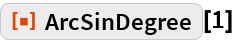Out=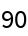Plot over a given range of values:

 In:=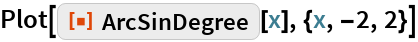Out=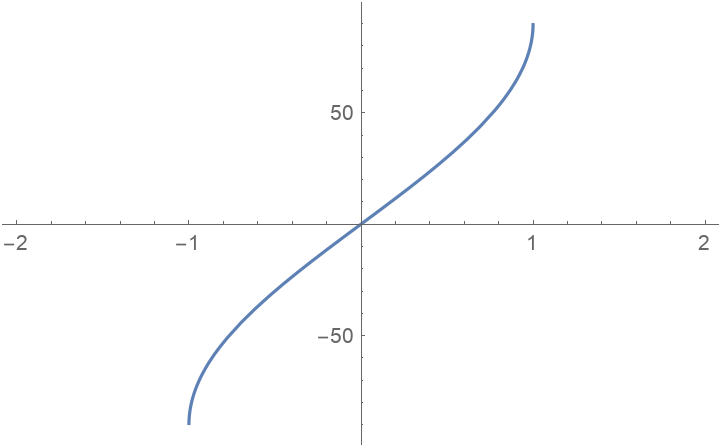### Scope

Evaluate numerically:

 In:=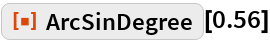Out=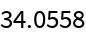Evaluate to high precision:

 In:=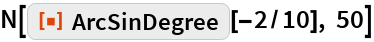Out=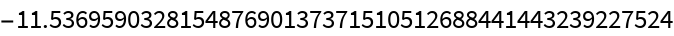## Requirements

Wolfram Language 11.3 (March 2018) or above